﻿ Educational Efficacy of Derivation Method for Partial Differential Equations in Thermodynamics

### Educational Efficacy of Derivation Method for Partial Differential Equations in Thermodynamics

Tetsuya Kanagawa, Hisao Taira

American Journal of Educational Research

## Educational Efficacy of Derivation Method for Partial Differential Equations in Thermodynamics

Tetsuya Kanagawa1,, Hisao Taira2

1Department of Engineering Mechanics and Energy, University of Tsukuba, Tsukuba 305-8573, Japan

2Department of Education, Hokkaido University of Education, Sapporo Campus, Sapporo 002-8502, Japan

### Abstract

One of the mathematical difficulties with regard to thermodynamics for undergraduate university students is the treatment of the total differential, which contains partial derivatives. The conventional treatment suppresses the derivation of the partial differential equations (e.g., energy equation and Mayer’s relation), reducing the student’s understanding of the physical aspects of thermodynamics. This paper proposes a systematic method for of deriving the partial differential equations in thermodynamics, based on both the total differential and the appropriate selection of independent variables of the thermodynamic state variables. To demonstrate our method, the energy equation and Mayer’s relation are derived via the systematic approach, and students’ misunderstandings concerning the derivation process are presented. The results of a questionnaire administered to evaluate student opinions indicate that our proposed method is effective.

• Tetsuya Kanagawa, Hisao Taira. Educational Efficacy of Derivation Method for Partial Differential Equations in Thermodynamics. American Journal of Educational Research. Vol. 5, No. 4, 2017, pp 362-366. http://pubs.sciepub.com/education/5/4/4
• Kanagawa, Tetsuya, and Hisao Taira. "Educational Efficacy of Derivation Method for Partial Differential Equations in Thermodynamics." American Journal of Educational Research 5.4 (2017): 362-366.
• Kanagawa, T. , & Taira, H. (2017). Educational Efficacy of Derivation Method for Partial Differential Equations in Thermodynamics. American Journal of Educational Research, 5(4), 362-366.
• Kanagawa, Tetsuya, and Hisao Taira. "Educational Efficacy of Derivation Method for Partial Differential Equations in Thermodynamics." American Journal of Educational Research 5, no. 4 (2017): 362-366.

 Import into BibTeX Import into EndNote Import into RefMan Import into RefWorks

### 1. Introduction

Mathematical expressions used to describe the laws of thermodynamics [1-6] are basically described by differential, i.e., infinitesimal, quantities. This is a remarkable feature compared to dynamics, electromagnetics, and so on, the laws of which are described by a differential coefficient as a finite quantity (e.g., velocity and pressure gradient) rather than the differential. The main purpose of thermodynamics is to determine the degree of variation of state variables when the thermodynamic state changes from one equilibrium state to another. Thermodynamic state variables are obtained by integrating an infinitesimal change in the state variables, which is represented as a total differential. Thus, one of the most important mathematical tools for thermodynamics is the total differential (equation) containing partial derivatives (not the partial differential) [7, 8].

During a thermodynamics lecture course presented by the first author (TK Lecture), it was found that undergraduate university students with the appropriate level of relevant education failed to understand the relationship between the total differential and the partial derivatives. In fact, many students could not use the differential operator symbols “d” and “∂” appropriately. Furthermore, many students could not understand that, although the total differential dx is an infinitesimal quantity, the partial derivative ∂f /∂x is a finite quantity. Note that the understanding of this distinction between finite and infinitesimal quantities is quite important for students with elementary knowledge. In fact, in an examination conducted during the TK Lecture, approximately 25 % of the examined students submitted the following equation as an answer: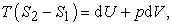(1)

where T, S, p, V, and U are the absolute temperature, entropy, pressure, volume, and internal energy, respectively. Further, the subscripts 1 and 2 denote the thermal equilibrium states. Obviously, Eq. (1) is incorrect, as the left- and right-hand sides of Eq. (1) feature finite and infinitesimal quantities, respectively, which thereby cannot be connected via the equal sign.

It was surprising to find this lack of fundamental mathematical understanding among many students. To understand thermodynamics, it is necessary to understand that state variables are functions of two variables that can be arbitrarily chosen; note that chemical reactions and material production are neglected and only single-component systems are considered in this paper, hence, the chemical potential and the treatment of three-variable function do not appear. The examination described above indicates that university students cannot fully employ this empirically known thermodynamic property, and thus, this situation requires improvement.

In this paper, we propose a systematic method for deriving partial differential equations, which is demonstrated via the derivation of two equations, i.e., energy equation and Mayer’s relation, as presented in Sections 3 and 4, respectively. We believe that the proposed method will comprehensively overcome students’ difficulty in understanding the relationship between the total differential and the partial derivatives. Furthermore, the proposed method fully utilizes the property that state variables are functions of two variables. The removal of this mathematical difficulty will facilitate greater student concentration on the physical aspects of thermodynamics. Evaluation results, in the form of student opinions regarding the proposed method, are presented in Section 3.6.

### 2. Research Group

Each year, the TK Lecture course, which comprises the “Thermodynamics I (two credits, spring semester)” and “Thermodynamics II (one credit, winter semester)” modules, is provided to approximately 80 students at the College of Engineering Systems, University of Tsukuba, Japan. This is a mandatory second-year course.

Students have previous knowledge of differentiation and integration, ordinary differential equations, linear algebra, classical mechanics, electromagnetics, and so on, which are taught in mandatory courses provided in the first year. The TK Lecture is regarded as an intermediate-level course between physical [1-3] and engineering thermodynamics [4-6], with engineering thermodynamics being covered by another, optional course.

### 3. Problem A: Energy Equation

3.1. Problem Statement

Let us consider the following problem: Derive the following energy equation for a quasi-statically reversible process(2)

In previous TK Lectures, the following instruction was given: “Write down the first law of thermodynamics.”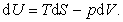(3)

TK then explained the process simply: “Divide both sides of Eq. (3) by dV under the assumption of an isothermal process. Then, you obtain Eq. (2). Through this simple process, the ordinary differential operator d disappears and the partial differential operator ∂ appears; this is natural as a mathematical rule. Note that all variables in Eqs. (2) and (3) are two-variable functions, i.e., the independent variables of U and S are V and T.”

Below, we refer to the abovementioned method as the “dividing method (DM).” In this paper, however, we propose an alternative method for deriving Eq. (2) using the total differential. The advantage of our method is that it removes the ambiguity regarding d and ∂. We refer to our proposed method as the “total differential method (TDM).” As shown in Section 4, TDM is also applicable to the derivation of other partial differential equations in thermodynamics, e.g., Mayer’s relation.

3.2. Student Misunderstanding

In an examination conducted as part of the TK Lecture, approximately 65 % of students obtained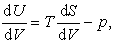(4)

in an attempt to derive Eq. (2) from Eq. (3). The use of d is quite incorrect, as U and S are two-variable functions. Such an incorrect answer is evidence that these students did not understand the basic difference between ordinary and partial differentials. Furthermore, we should recognize that the partial differential operator ∂/∂V and the partial derivative ∂U/∂V should be treated as one item in Eq. (2). We also should note that the ordinary differential operator d/dV, the numerator (total differential) dU, and the denominator (total differential) dV should be treated as one item on the left-hand side of Eq. (4). In particular, the rigorous treatment of ordinary and partial differentials is extremely important in thermodynamics. For example, many students could not distinguish between the partial derivative appearing as a finite quantity in Maxwell’s relation [Eqs. (14) and (27) below] and the total differential dU in the first law of thermodynamics [Eq. (3)].

In the abovementioned lectures, TK explained that “The change from d to ∂ is natural” and that “U and S are functions of two variables.” However, students are rarely satisfied with these explanations. Although the operation of division by dV is frequently used in thermodynamics, its validity has not been explained in detail. Thus, a precise and rigorous treatment is required.

Why does the differential operator d change to ∂ in the derivation process from Eq. (3) to Eq. (2)? It is difficult to present an effective explanation for this change to university students who are only beginning to learn the difference between ordinary and partial differentials. In fact, questions regarding the difference between d and ∂ have been extensively raised during the TK Lecture course. Therefore, from the perspective of physics education and to avoid confusion, the DM approach should be avoided. The alternative TDM approach, which is presented in the following section, has been found to be extremely effective as described in Section 3.6 below. The essence of the proposed TDM is that there is no reliance on division by dx.

3.3. Revised Lecture Featuring TDM

Focusing on dS and dV on the right-hand side of Eq. (3), we discover the use of the total differential. We determine the independent variables of U as S and V, yielding U = U(S, V). Thus, the total differential dU (S, V) is written as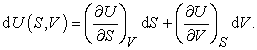(5)

In this case, the left-hand side of Eq. (3) is dU = dU(S, V) and Eq. (5) should be satisfied for arbitrary dS and dV; thus, we have(6)

Because Eq. (5) contains three variables—U, S, and V—we define S = S(V,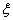) in U(S, V), where the new independent variableis an arbitrary variable for generality, which may be set to p or T, for example. The total differential dS(V,) is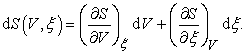(7)

Substituting the right-hand side of Eq. (7) into dS in the first term on the right-hand side of Eq. (5), we obtain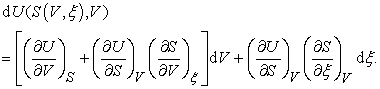(8)

Here, the treatment of the independent variables is important, i.e.,(9)

with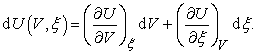(10)

That is, the right-hand side of Eq. (8) can be treated as an identity with respect to dV and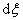, and we thus obtain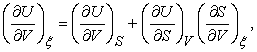(11)(12)

respectively. Note that Eqs. (11) and (12) are frequently utilized in the derivation of partial differential equations in thermodynamics. We emphasize that they are derived from a thermodynamics-based discussion; that is, we employ the property that the thermodynamic state variables are two-variable functions.

Finally, substituting Eq. (6) into the right-hand side of Eq. (11) yields(13)

which conforms to Eq. (2) for the case= T. In this manner, Eq. (2) can be derived via systematic utilization of the total differential. Thus, it is important to distinguish between the total differential and the partial differential of a thermodynamic variable.

3.4. Maxwell’s Relation and Joule’s Law

Substituting Maxwell’s relation(14)

into the first term on the right-hand side of Eq. (13) for the case= T, we obtain(15)

We then assume that the thermodynamic system is an ideal gas. Further, the substitution of the equation of state for an ideal gas reveals that the right-hand side of Eq. (15) is equivalent to zero, i.e.,(16)

Finally, we can derive Joule’s law, i.e., the fundamental and important relation for internal energy: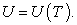(17)
3.5. Student Hesitation: Deletion of Entropy

Although the aforementioned calculations are straightforward and simple, many students struggle to accept the substitution of Maxwell’s relation given in Eq. (14) into Eq. (13). We assume that this difficulty arises because many students intensively focus on the mathematical aspect of this derivation. As is clear from Section 3.4, the importance of this substitution is the deletion of S only, which TK repeatedly emphasized: “You must delete S!”. As a result, approximately 90 % of students understood the importance of eliminating S.

An expert in thermodynamics easily performs the deletion of S, as S is unclear. However, many undergraduate students do not want to eliminate S. In fact, a student stated as follows: “I cannot conceive of an operation to delete the new variable S, because I want to discover the meaning of S.” We believe that many students are enchanted by the mysterious concept of entropy and desire to elucidate the details of the entropic behavior encountered in thermodynamics. Therefore, they hesitate to delete an important variable such as S, as this operation is quite different compared to the mathematics employed in other branches of physics.

3.6. Evaluations by Students

In the revised TK Lecture “Thermodynamics II (winter semester, fiscal year 2016),” TK introduced both TDM and DM to 74 students (see also Section 2). In order to determine which method is easier to understand, TK supplied a questionnaire to every student. The questionnaire posed the question: “Is it easier for you to understand TDM compared to DM?” The answer sheet contained three check boxes, corresponding to the responses, “more difficult to understand,” “the same,” and “easier to understand.” In addition, a space was provided for students to explain their opinions.

A total of 58 (approximately 80 %) students checked “easier to understand.” The common student opinions were as follows:

(i) TDM is easier to understand than DM, because the operator ∂ naturally appears;

(ii) Although many straightforward calculations are required in TDM than in DM, TDM has removed my ambiguity surrounding this derivation;

(iii) I had not previously considered the physico-mathematical meaning of and difference between d and ∂ in depth. By focusing on this concept in detail, I developed an understanding of the essential and remarkable difference between these symbols. In addition, I now recognize that it is important to focus on rigorous and precise expressions in mathematics for thermodynamics.

Based on these student opinions, we believe that many students simply wish to eliminate the ambiguity inherent to the DM rather than to avoid straightforward calculations. We therefore conclude that our proposed TDM is easy to follow, and it facilitates deeper understanding of the relevant concepts.

### 4. Problem B: Mayer’s Relation

In this section, to demonstrate the applicability of TDM, we derive Mayer’s relation as another example of a relevant partial differential equation.

4.1. Derivation

Let us begin with the difference of the isobaric and isomeric heat capacities, respectively, CP and CV, which is described as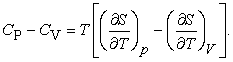(18)

The first and second terms on the right-hand side of Eq. (18) indicate the appropriate choice of dependence of the independent variables, i.e., S(T, p) and S(T, V), respectively. Then, we write down the total differentials:(19)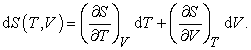(20)

As four variables appear in Eqs. (19) and (20), i.e., S, T, p, and V, we wish to reduce the three differentials on the right-hand sides, dT, dp, and dV, to two differentials. Thus, we further assume the following dependence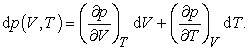(21)

Substituting Eq. (21) into the second term on the right-hand side of Eq. (19) gives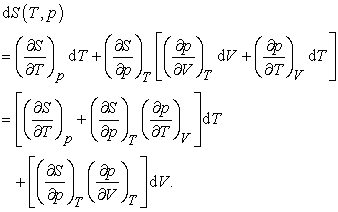(22)

In the same manner as in Eq. (9), we note the transition of independent variables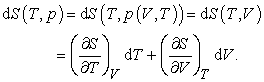(23)

That is, the choice of independent variables of S, (T, p) naturally varies to (T, V). Comparison of the coefficients of dT and dV in the right-hand side of Eqs. (22) and (23) gives(24)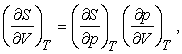(25)

respectively. We repeatedly emphasize that the above formula for partial derivatives appears naturally when discussing thermodynamics.

Substituting Eq. (24) into the second term on the right-hand side of Eq. (18), we obtain(26)

Furthermore, substituting Maxwell’s relation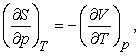(27)

into Eq. (26) yields Mayer’s relation:(28)
4.2. Student Errors and Improvements

Many students exhibited a misunderstanding in the TK Lecture. That is, with respect to Eq. (18), many students supplied the incorrect response(29)

This is quite the misunderstanding. Furthermore, approximately 50 % of students could not combine Eqs. (19), (20), and (21). Such a student tended to fail a final examination of the TK Lecture course.

These difficulties are caused by the arbitrariness of the independent variable selection. Therefore, with the aim of improving the efficacy of these thermodynamics lectures, we must repeatedly emphasize the importance of choice regarding the selection of independent variables. No correct selection exists. In the TK Lecture, every student can now discover a tool for the selection of independent variables.

### 5. Conclusions

The ordinary differential operator d sometimes changes to the partial differential operator ∂ during the derivation of the mathematical expressions for the laws of thermodynamics. Although this operation is frequently seen, it is also a critical source of ambiguity for undergraduate university students.

In this paper, we proposed TDM, i.e., a derivation method based on the total differential that eliminates this source of ambiguity for students. The main results are summarized as follows:

(i) Focusing on the treatment and selection of independent variables, systematic utilization of the total differential is achieved. Consequently, division by infinitesimal quantities can be avoided in our proposed systematic derivation method for partial differential equations.

(ii) The appropriate selection of independent variables is one of the most difficult tasks faced by undergraduate university students. In TDM, the independent variables are naturally and systematically determined.

(iii) TDM is based on one of the most important principles of thermodynamics: The selection of independent variables is arbitrary. This generates a focus on the physical viewpoint rather than the mathematical perspective, in contrast to DM.

Quantitative evaluation of the effect of this revised educational approach will be reported in a forthcoming paper. We hope that our method will be utilized extensively thermodynamics lectures in future.

### Statement of Competing Interests

The authors declare no competing interests.

### References

  Fermi, E., Thermodynamics (Dover Books on Physics), Dover Publications, New York, 1956.In article  Callen, H.B., Thermodynamics and an Introduction to Thermostatistics, Wiley, New York, 1985.In article  Hill, T.L., An Introduction to Statistical Thermodynamics (Dover Books on Physics), Dover Publications, New York, 1987.In article  Reynolds, W.C. and Perkins, H.C., Engineering Thermodynamics (2nd Edition), McGraw-Hill, New York, 1977.In article  Cengel, Y. and Boles, M., Thermodynamics: An Engineering Approach (8th Edition), McGraw-Hill, New York, 2014.In article  Moran, M.J., Shapiro, H.N., Boettner, D.D. and Bailey, M.B., Fundamentals of Engineering Thermodynamics (8th Edition), Wiley, New York, 2014.In article  Poisson, E., Statistical Physics I, Lecture Notes (Fall 2000), Department of Physics, University of Guelph [Online] Available: https://www.physics.uoguelph.ca/~epoisson/research/spi.pdf/ [Accessed Mar. 28, 2017].In article  Wang, C.Y. and Hou, C.H., “Teaching Differentials in Thermodynamics Using Spatial Visualization,” Journal of Chemical Education, 89 (12), 1522-1525, 2012.In article View Article